# Shower Temperature Sensor

Using this setup, we will successfully measure shower temperature and alert the user that the shower is ready.

IntermediateFull instructions provided20 hours2,007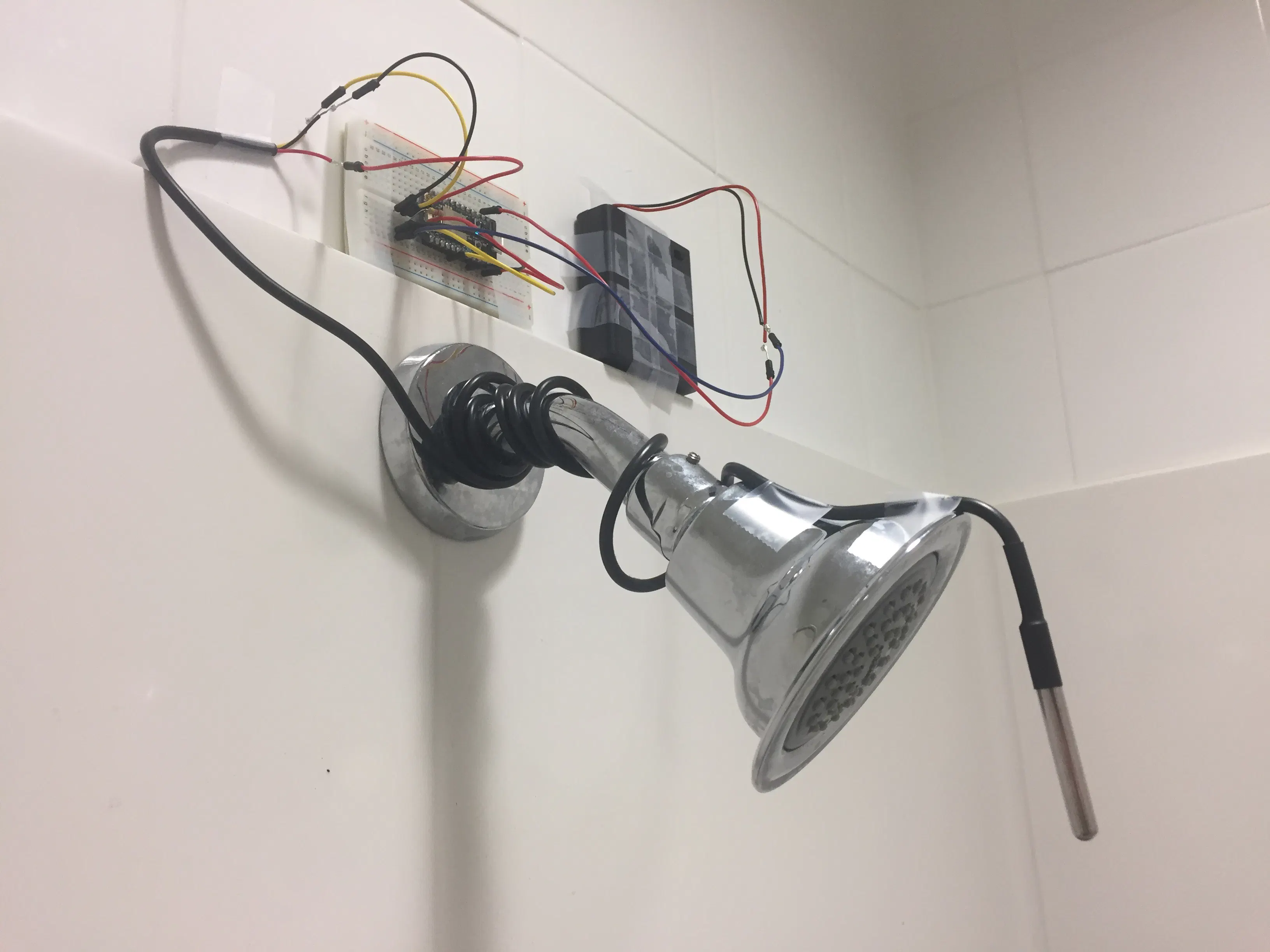## Things used in this project

### Hardware componentsParticle Photon
×2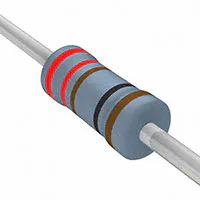Resistor 221 ohm
×3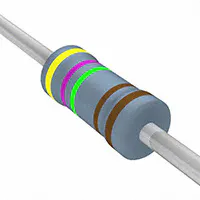Resistor 4.75k ohm
×1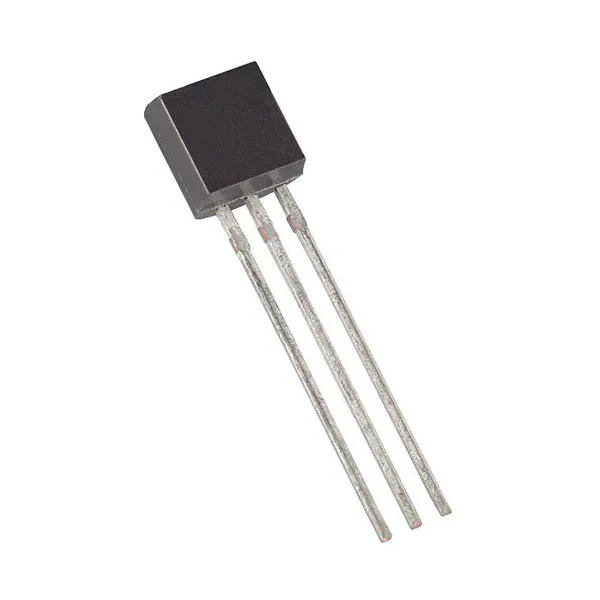Maxim Integrated DS18B20 Programmable Resolution 1-Wire Digital Thermometer
×1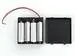4xAA battery holder
×1LED (generic)
×3×2

### Software apps and online services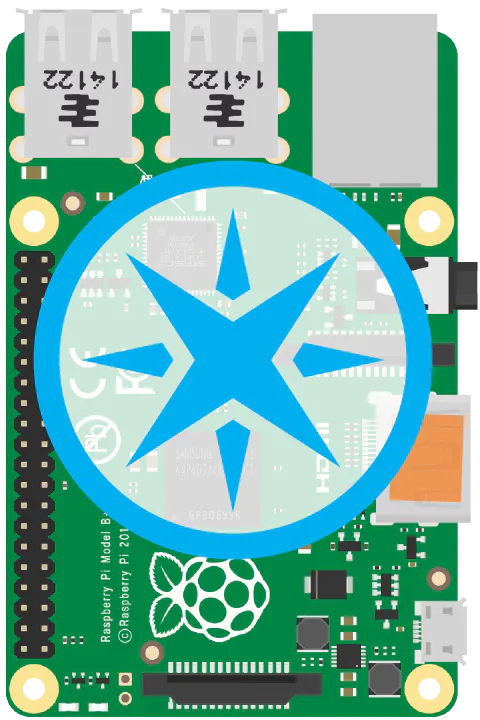Particle Pi

## Schematics

### Circuit Diagrams

These two images show the circuit diagrams for both photon boards.

## Code

### Indicator Code

C/C++
This code was uploading to the second board. It receives an input from the first board and lights one of three LEDs. In addition, when receiving data, it sends a signal back to the first particle board to light the D7 LED.
```float V = 1;
int led2 ;
void setup() {
pinMode(D0, OUTPUT);
pinMode(D1, OUTPUT);
pinMode(D2, OUTPUT);
Particle.subscribe("Light_1", Light, MY_DEVICES);
}
void Light(const char *event, String data) {
led2=data.toInt();
if(led2== 2){
digitalWrite(D0, HIGH);
digitalWrite(D1, LOW);
digitalWrite(D2, LOW);}
else if(led2== 3){
digitalWrite(D1, HIGH);
digitalWrite(D0, LOW);
digitalWrite(D2, LOW);}
else if(led2== 4){
digitalWrite(D2, HIGH);
digitalWrite(D0, LOW);
digitalWrite(D1, LOW);}
else{
digitalWrite(D0, LOW);
digitalWrite(D1, LOW);
digitalWrite(D2, LOW);}
String connect = String(V);
Particle.publish("connect_1", connect, PRIVATE);

}
```

### Temperature Sensor Code

C/C++
This code allows the temperature sensor unit to detect the temperature, determine if the water is too hot or cold, determine the connection of the system and publish the temperature to adafruit.io.

Approximately half of the code came from Particle's "Community Libraries". Specifically, the "OneWire" and "Adafruit_MQTT" libraries. Additionally some of the code pertaining to Adafruit originated from "MEGR 3171: adafruit example.txt" found on the MEGR 3171 canvas page. Finally the code to interpret the data from the temperature sensor was acquired from the particle.io tutorial "Tutorial #4: Temperature Logger".
```// This #include statement was automatically added by the Particle IDE.
#include <OneWire.h>
OneWire ds = OneWire(D4);
unsigned long lastUpdate = 0;
float lastTemp;
void setup() {
Serial.begin(9600);
pinMode(D3, OUTPUT);
pinMode(D5, OUTPUT);
digitalWrite(D3, LOW);
digitalWrite(D5, HIGH);

}
#define AIO_SERVERPORT  1883                   // use 8883 for SSL
#define AIO_KEY         "046fc1faa19340dfa922d67f068ec31c"
TCPClient TheClient;
void loop(void) {
byte i;
byte present = 0;
byte type_s;
byte data;
float celsius, fahrenheit;
Serial.println();
ds.reset_search();
delay(250);
return;
}
Serial.print("ROM =");
for( i = 0; i < 8; i++) {
Serial.write(' ');
}
Serial.println("CRC is not valid!");
return;
}
Serial.println();
case 0x10:
Serial.println("  Chip = DS1820/DS18S20");
type_s = 1;
break;
case 0x28:
Serial.println("  Chip = DS18B20");
type_s = 0;
break;
case 0x22:
Serial.println("  Chip = DS1822");
type_s = 0;
break;
case 0x26:
Serial.println("  Chip = DS2438");
type_s = 2;
break;
default:
Serial.println("Unknown device type.");
return;
}
ds.reset();
ds.write(0x44, 0);
delay(1000);
present = ds.reset();
ds.write(0xB8,0);
ds.write(0x00,0);
present = ds.reset();
ds.write(0xBE,0);
if (type_s == 2) {
ds.write(0x00,0);
}
Serial.print("  Data = ");
Serial.print(present, HEX);
Serial.print(" ");
for ( i = 0; i < 9; i++) {
Serial.print(data[i], HEX);
Serial.print(" ");
}
Serial.print(" CRC=");
Serial.print(OneWire::crc8(data, 8), HEX);
Serial.println();
int16_t raw = (data << 8) | data;
if (type_s == 2) raw = (data << 8) | data;
byte cfg = (data & 0x60);
switch (type_s) {
case 1:
raw = raw << 3;
if (data == 0x10) {
raw = (raw & 0xFFF0) + 12 - data;
}
celsius = (float)raw * 0.0625;
break;
case 0:
if (cfg == 0x00) raw = raw & ~7;  // 9 bit resolution, 93.75 ms
if (cfg == 0x20) raw = raw & ~3; // 10 bit res, 187.5 ms
if (cfg == 0x40) raw = raw & ~1; // 11 bit res, 375 ms
// default is 12 bit resolution, 750 ms conversion time
celsius = (float)raw * 0.0625;
break;
case 2:
data = (data >> 3) & 0x1f;
if (data > 127) {
celsius = (float)data - ((float)data * .03125);
}else{
celsius = (float)data + ((float)data * .03125);
}
}
if((((celsius <= 0 && celsius > -1) && lastTemp > 5)) || celsius > 125) {
celsius = lastTemp;
}
fahrenheit = celsius * 1.8 + 32.0;
lastTemp = celsius;
Serial.print("  Temperature = ");
Serial.print(celsius);
Serial.print(" Celsius, ");
Serial.print(fahrenheit);
Serial.println(" Fahrenheit");
String temperature = String(fahrenheit);
Particle.publish("temperature", temperature, PRIVATE);
delay(10000);
}
```

## Credits

### Kyle Tucker

1 project • 0 followers
Mechanical Engineering Student at the University of North Carolina at Charlotte.

### Dylan Duplessis

0 projects • 0 followers